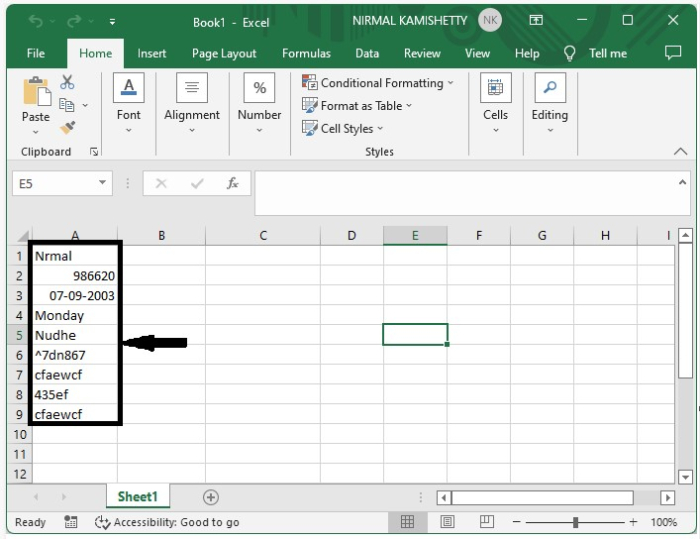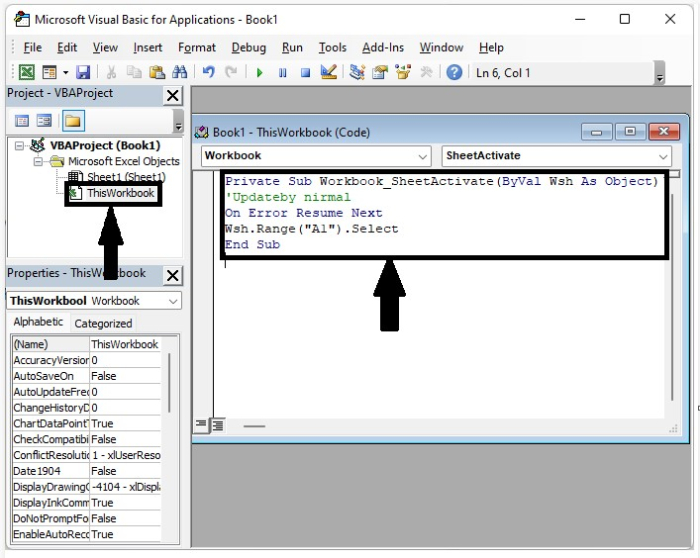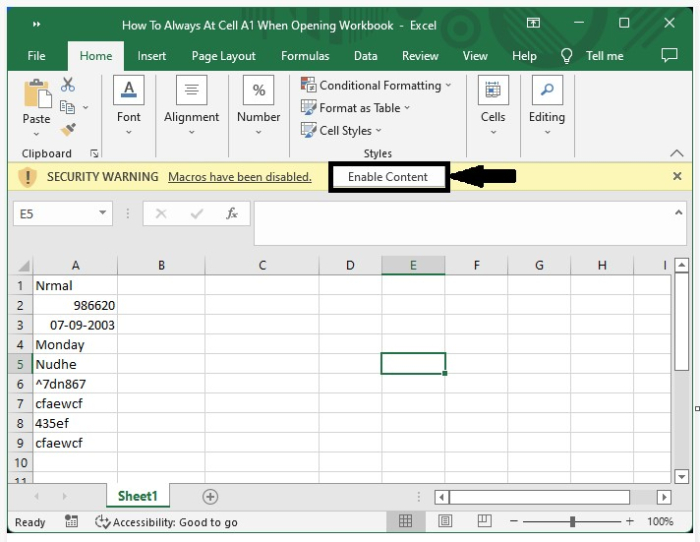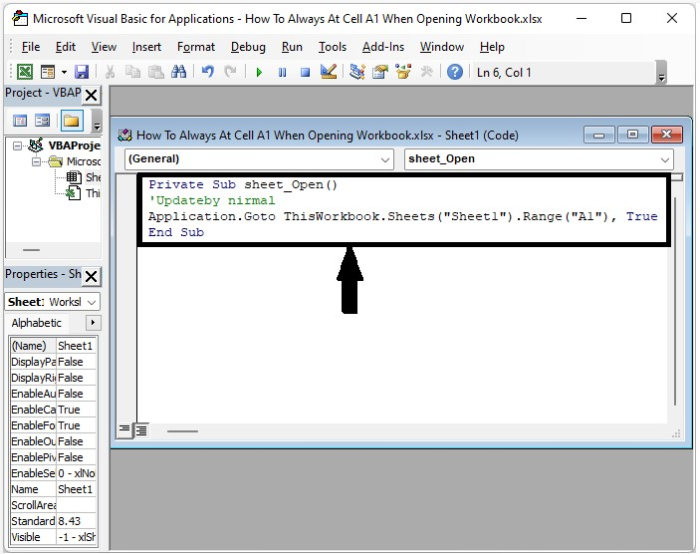# How to Start at Cell A1 Always when Opening an Excel Workbook?

When we open a new excel sheet, we can see that the sheet will start from the cell A1, but when we open an existing worksheet, we can see that the sheet will start from where we last saved it. When we send the sheet to other people, they will be able to know where you last edited, but they will not be able to know if it starts in cell A1.

This tutorial will help you understand how we can always open a workbook at cell A1. Here, we will have two parts: first, the code will work for the whole workbook, and the second part will only work for the specified sheet in the workbook.

## Always Start at Cell A1 when Opening a Workbook for All Sheets

Here we will add VBA code to the whole workbook to complete our task. Let us see a simple process to see how we can open a workbook with cell A1 for all sheets in the workbook.

Step 1

Consider an Excel sheet with data similar to the data shown in the image below.Now use the command ALT+F11 to open the VBA, double-click on this workbook, and enter Program 1, as shown in the below image.

### Example 1

Private Sub Workbook_SheetActivate(ByVal Wsh As Object)
'Updateby nirmal
On Error Resume Next
Wsh.Range("A1").Select
End SubStep 2

Now close the VBA and save the excel sheet by clicking on "file as VBA enabled." Open the sheet and click on "enable code" to start at cell A1.## Always Start at Cell A1 when Opening a Workbook for a Specific Sheet

Here we will add VBA code to a single sheet. Let us see a simple process to always open at cell A1 when opening a workbook for a specific sheet in the workbook.

Let us consider the same data that we used in the above example and use the command ALT+F11 to open the VBA, double-click on this workbook, and enter Program 2, as shown in the below image.

### Example 2

Private Sub sheet_Open()
'Updateby nirmal
Application.Goto ThisWorkbook.Sheets("Sheet1").Range("A1"), True
End SubNow save the Excel file with VBA enabled and click on enable content, as we did in the above example.

## Conclusion

In this tutorial, we used a simple example to demonstrate how we can always select cell A1 when opening a workbook in Excel to highlight a particular set of data.

Updated on: 16-Jan-2023

757 Views